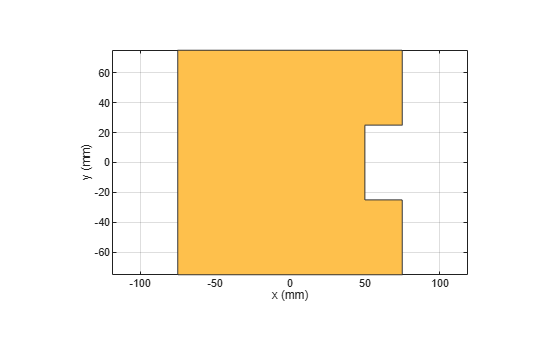# area

Calculate area of shape in square meters

## Syntax

``a = area(shape)``

## Description

example

````a = area(shape)` calculate area of the shape in units sq.m.```

## Examples

collapse all

Create a rectangle with a length of 0.15 m, and a width of 0.15 m.

`r = antenna.Rectangle('Length',0.15,'Width',0.15);`

Create a second rectangle with a length of 0.05 m, and a width of 0.05 m. Set the center of the second rectangle at half the length of the first rectangle r.

`n = antenna.Rectangle('Center',[0.075,0],'Length',0.05,'Width',0.05);`

Create and view a notched rectangle by subtracting n from r.

```rn = r-n; show(rn)```Calculate the area of the notched rectangle.

`area(rn)`
```ans = 0.0212 ```

## Input Arguments

collapse all

Shape created using custom elements and shape objects of Antenna Toolbox™, specified as an object.

Example: `c = area(rectangle)` where rectangle is created using `antenna.Rectangle` object.

## Version History

Introduced in R2017a